# A New Approach to Simulate Stirling Engine Regenerators as Porous Media under Low Reynolds Conditions

A New Approach to Simulate Stirling Engine Regenerators as Porous Media under Low Reynolds Conditions
School of Engineering, University of Basilicata, Potenza 85100, Italy

Env. and Applied Fluid Dynamics Dept., von Karman Inst. for Fluid Dynamics, Sint-Genesius-Rode, B-1640, Belgium

Corresponding Author Email:
maria.faruoli@unibas.it
Page:
958-965
|
DOI:
https://doi.org/10.18280/ijht.370404
25 October 2019
|
Accepted:
5 December 2019
|
Published:
26 December 2019
| Citation

OPEN ACCESS

Abstract:

The regenerator of a Stirling engine is widely studied both numerically and experimentally because it greatly influences the global performance of such an engine. In this work, a new numerical approach is developed to analyse the regenerator performance. As in conventional porous media models, this approach consists of defining the wires of the regenerator as regions with a high value of inverse permeability. On the other hand, a lower porosity value is used in the boundary layers and additional terms are introduced in the governing equations to correctly reproduce the flow structure and the overall regenerator performance. This approach leads to a very interesting reduction of the computational cost of the simulations w.r.t. standard CFD analyses. OpenFOAM libraries are used and low Reynolds number conditions (Re lower than 200) are considered. At first, it is shown that at low Re the incompressible flow hypothesis is accurate enough to predict fluid-dynamic and thermal performances of the regenerator. Hence, the proposed porous media model has been implemented by considering the fluid as incompressible. The model is applied to a test case under several flow conditions and the results are compared with those obtained by the standard CFD incompressible model, showing a good agreement for both the friction coefficient and the thermal efficiency.

Keywords:

porous media, CFD, regenerator, Stirling engine

1. Introduction

Stirling engine is a highly reliable external combustion engine, based on a regenerative work cycle. The use of air as working fluid makes this type of engine very attractive for industrial applications. Moreover, a large variety of heat sources can be used to run the engine. The drawbacks of these sources, however, are their low specific power and relatively slow start.

Great attention is focused on the regenerator of such engines, i.e. the heat exchanger placed between the hot and the cold streams. It is mainly intended to store the heat from the hot stream and to transfer the recovered heat to the cold stream. By optimizing this process, a great improvement of the engine efficiency can be achieved. This component represents a part of the Stirling engine that characterizes it with respect to other external combustion engines, and the regenerator performances greatly influence the global efficiency of the power system . Tanaka et al.  have analysed the flow field in a regenerator under laminar and oscillating flow conditions and they have shown that the regenerator efficiency can reach extremely high values, even close to unity, under specific flow conditions.

Among the others, the stacked woven wires regenerator type represents one of the most studied. The wires net may have different configurations, optimized to obtain the best performance in terms of both aerodynamics and thermal efficiency. The objective of the design process is to maximize the heat transfer between the wires and the flow while keeping low the pressure losses through the net.

Several recent works in the scientific literature are concerned with Stirling engines, in order to exploit their advantages. Both experimental and numerical analyses have been performed. The experimental configuration used by Gheith et al.  is the Stirling Test Bench Regenerator (STBR), which consists of an apparatus to test the regenerator at high pressure under periodic oscillating flow in both directions of the piston stroke. In the work of Costa et al. , different parameters of a regenerator with γ configuration are analysed with the aim of improving the thermal efficiency. From a numerical point of view, two main approaches are generally employed. In the first approach, the wires are modelled as solid walls, and the fluid flow through the regenerator is studied by means of multi-dimensional Computational Fluid Dynamics (CFD), as reported by Costa et al. [5, 6], Faruoli et al.  and Bello et al. . Generally, the friction coefficient, the thermal efficiency and the Nusselt number are computed to assess the fluid-dynamic and the thermal performances of the regenerator. In the second approach, the wires matrix volume is approximated as a unique porous block, whose porosity value is defined in order to reproduce the overall fluid-dynamic behaviour of the flow through the regenerator. The computational cost of the simulations based on this method is reduced with respect to the first approach, due to the use of a structured computational grid. However, a drawback of this method is that the details of the geometry are not simulated, so some features of the flow field are lost. This approach has already been used to analyse heat exchangers  and, more specifically, it has been applied to a Stirling engine regenerator [1, 10, 11].

The main objective of this work is the development of a new numerical approach to model the engine regenerator as porous media. An investigation of the flow structure through the regenerator and the description of this new numerical model  will be given in the next sections.

Specifically, in the first part of this work, simulations with both incompressible and compressible assumptions have been performed in order to analyse the differences in the results of the two computed flow fields. This comparison has shown that the incompressible assumption can be used without losing accuracy in the evaluation of pressure losses and thermal efficiency. Moreover, the incompressible assumption allows a simplification of the model, leading to a reduction of the overall computational cost.

In the second part of this work, the new numerical approach is described and several simulations are carried out. The proposed numerical strategy simulates the wires as fully solid zone, by setting a high value of the inverse permeability in the numerical cells of the domain where the wires are placed, whereas lower values are used in the boundary layers. By employing this strategy, it is possible to both analyse the details of the flow field and compute the global parameters used to assess the regenerator performances. Moreover, this model can be used as a starting point for a topological optimization procedure . Finally, in the last part of this paper the model is validated by comparing it with a standard CFD approach under the incompressibility assumption. The regenerator configuration described by Bello et al.  is used for such an analysis.

2. Incompressible Model

2.1 Computational domain

The Stirling engine regenerator is composed of misaligned wire netting modules. The optimal configuration in the work of Bello et al.  is used. The modules are assembled one next or on top of each other. In this work, one single module is considered.

## 1.pngFigure 1. Regenerator matrix configuration and computational domain

The computational domain is defined as a parallelepiped with a cross-section of 1 mm x 1 mm and a total length of 5 mm, as shown in Figure 1. The wires module is located 2 mm downstream the inlet section. The regenerator is characterized by the following geometric parameters: the length, L, the hydraulic diameter, dh, and the porosity, πv, whose values are summarized in Table 1. The regenerator porosity is computed as defined in Eq. (1) and it is used to compute the hydraulic diameter, given in Eq. (2):

$\pi_{v}=\frac{V_{t o t}-V_{m}}{V_{t o t}}$, (1)

$d_{h}=\frac{4 \pi_{v}}{\varphi\left(1-\pi_{v}\right)}$. (2)

In the above equations, Vtot is the total volume of the matrix and Vm is the volume of the wires, Vtot is the ratio between the surface area and the volume of the woven matrix.

Table 1. Regenerator geometric specifications

 L[m] dh[m] πv 0.922 0.172 0.641

2.2 Mathematical model

In previous works [7, 8], the flow field has been simulated by assuming the fluid as compressible and thermal phenomena are taken into account by evaluating the heat fluxes between fluid and wires. A non-monotonic behaviour of the friction coefficient versus Reynolds number, Re, is observed, due to the compressibility effects. Indeed, such effects are not negligible above $R e \approx 200$ . The friction coefficient decreases with increasing Re, in the low Re regime, whereas it increases after $R e \approx 200$, due to the change in density. In this section, the hypothesis of negligible compressibility effects with low Re is proven to be valid for both the fluid-dynamic and the thermal behaviour of the fluid flow. Hence, a numerical model with constant fluid properties is considered and the temperature distribution in the domain is modelled by adding the energy equation. The incompressible results are compared with those obtained by including the fluid compressibility . Since a transition from laminar to turbulent regime is observed around $R e \approx 500$, the flow conditions investigated, with Re lower than 200, correspond to a laminar regime.

The governing equations are the Navier-Stokes (NS) continuity (Eq. (3)), momentum (Eq. (4)) and energy (Eq. (5)) equations for incompressible flow:

$\nabla \cdot \boldsymbol{u}=0$,     (3)

$\boldsymbol{u} \cdot \nabla \boldsymbol{u}-\nabla \cdot(v \nabla \boldsymbol{u})+\nabla p=0$,             (4)

$(\rho c) \boldsymbol{u} \cdot \nabla T-\nabla \cdot[k \nabla T]=0$,            (5)

where, $u$, $p$ and T are the fluid velocity, pressure and temperature, respectively; v is the fluid kinematic viscosity, $\rho$ is the fluid density, c and k the fluid thermal capacity and conductivity, respectively. The thermal physical properties of air at 20°C are used (Table 2) for the working fluid.

Table 2. Physical properties of fluid material

 Density [kg/m3] Specific Heat [J/(kg K)] Thermal Conductivity [W/(m K)] 1.17 1004.9 0.026

The same numerical grid used by Faruoli et al.  has been employed to perform all the simulations.

2.3 Boundary conditions

Simulations have been performed by employing a uniform velocity profiles at the inlet section with different magnitudes, as summarized in Table 3, together with the corresponding Reynolds number, defined as:

$R e=\frac{\rho u_{\max } d_{h}}{\mu}$,                      (6)

where, $u_{\max }=u_{\text {in}} / \pi_{v}$ and $\mu$ is the dynamic viscosity of the fluid.

Pressure at the outlet section is set equal to 1 bar. In this work it is assumed that heat is transferred from the hot stream to the wires, so the inlet temperature is set equal to 500 K, whereas the temperature of the wires is equal to 300 K.

Table 3. Inlet velocity conditions and corresponding Re

 Re 7.84 24.61 60.11 92.08 141.46 185.6 uin [m/s] 0.64 2 4.88 7.49 11.5 15.09

2.4 Results

The fluid-dynamic and the thermal performance of the regenerator are evaluated by using a friction coefficient to compute the pressure losses thru the matrix and the spatially-averaged temperature at the outlet section. The friction coefficient is computed as:

$c_{f}=\frac{\Delta p}{\frac{1}{2} \rho u_{\max }^{2}}$,                (7)

where, $\Delta p=p_{i n}-p_{o u t}$.

The temperature difference between outlet and inlet can be used as a measure of the amount of heat transferred from the fluid to the wires. Thus, a higher temperature difference corresponds to a higher efficiency of the heat exchanger. The difference is normalized w.r.t. the difference between wires and inlet temperature, by using the following definition:

$\phi=\frac{T_{o u t}-T_{i n}}{T_{\text {wires}}-T_{i n}}$.                    (8)

A value of $\phi$ close to unity corresponds to the highest efficiency.

Figure 2 shows the dependence of the friction coefficient, $c_{f}$, on the Reynolds number, Re, under the incompressible assumption and a comparison with the results obtained by using the compressible flow assumption . The figure shows that a maximum difference of about 5% occurs at very low Re.

The velocity distribution normalized w.r.t. the maximum velocity in the domain in the case Re=84 is shown in Figure 3 for both compressible and incompressible assumptions. For the compressible simulation, the fluid velocity through the first row of wires is higher than the velocity through the third row. Instead, in the case of incompressible flow, smaller differences are observed. This difference is mainly due to the fluid density since it varies from 0.7 to 1.2 kg/m3 under the compressibility assumption, as shown in Figure 4.

In Figure 5, the dimensionless pressure profiles, defined as:

$\bar{p}=\frac{p}{\frac{1}{2} \rho v_{\max }^{2}}$,                (9)

is shown along the centerline, for Re=84. The figure shows very similar profiles, with some small differences.

## 2.png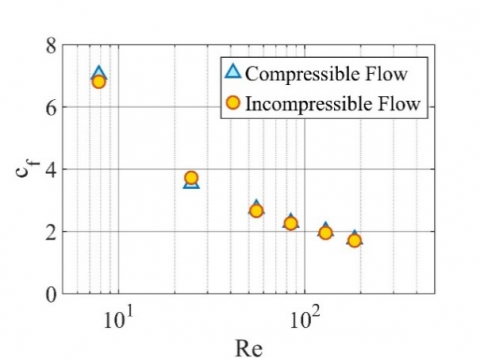Figure 2. Comparison between compressible and incompressible fluid assumption, $c_{f}-\mathrm{Re}$, Tin=500 K and Twall=300 K

## 3.png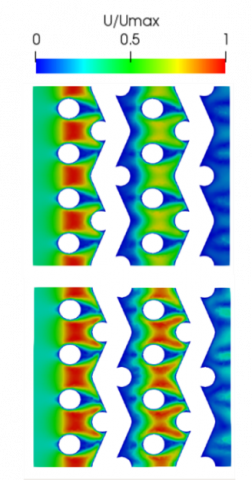Figure 3. Comparison between compressible (top) and incompressible (bottom) fluid assumption, normalized velocity on YZ middle plane, Tin=500 K and Twall=300 K, Re=84

## 4.pngFigure 4. Density contour plot on YZ middle plane of the wires matrix for compressible fluid flow, Tin=500 K and Twall=300K, Re=84

## 5.png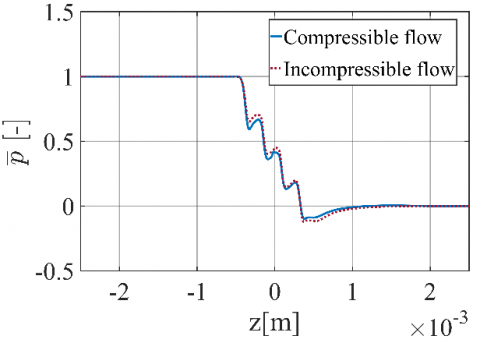Figure 5. Comparison between compressible and incompressible fluid assumption, dimensionless pressure profile along z-direction, Tin=500 K and Twall=300 K, Re=84

## 6.png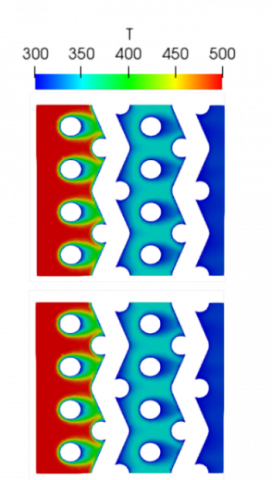Figure 6. Comparison between compressible (top) and incompressible (bottom) fluid assumption, temperature contour plots on YZ middle plane, Tin=500 K and Twall=300 K, Re=84

## 7.pngFigure 7. Comparison between compressible and incompressible fluid assumption, temperature profiles along z-direction, Tin=500 K and Twall=300 K, Re=84

Even for the temperature distribution, very similar temperature fields (Figure 6) and temperature profiles along the centerline (Figure 7) have been observed. This is due to a small influence of the density variation on the thermal results. These small differences result in a good agreement in terms of thermal efficiency, with a maximum difference of 3%, as shown in Figure 8, where the thermal efficiency vs Re is given.

Based on such results, the incompressible flow assumption can be considered as a valid approach within the Re range from 5 to 200, in order to compute the overall efficiency of the regenerator, despite the differences in the velocity distribution. Moreover, the use of the incompressible assumption allows to considerably reduce the overall computational cost of the simulations. Hence, the incompressible assumption is also used in the porous media model described in the following section.

## 8.pngFigure 8. Comparison between compressible and incompressible fluid assumption, $\phi$-Re, Tin=500 K and Twall=300 K

3. Porous Model

3.1 Computational domain

In this section, a new approach is presented in order to study the thermo-fluid dynamic peculiarities of the flow through the engine regenerator. The numerical domain is a parallelepiped with the same dimensions given in the previous section. In this case, the geometry of the domain is simplified by considering the wires as zones of porous material. In each computational cell the porosity is defined as the fluid volume fraction, $\varepsilon$, given by the following equation:

$\varepsilon=\frac{\text {volume of fluid}}{\text { total volume }}$.              (10)

## 9.pngFigure 9. Porosity distribution with the artificial boundary layer

The numerical cells located within the wires are characterized by a value of $\varepsilon=0$, corresponding to a fully solid zone, whereas the remaining numerical cells are characterized by a value of $\varepsilon=1$, which defines a fully fluid zone. Unfortunately, this strategy does not allow any interaction between solid and fluid zones, thus no boundary layers are provided. Hence, to obtain an accurate simulation of the flow behaviour, an artificial boundary layer has been introduced. Such a layer is defined by using an intermediate value of the solid fraction evaluated by means of a parametric analysis. Figure 9 shows the ε distribution for the different zones including the artificial boundary layer, i.e. ε = 0.2.

3.2 Mathematical model

The flow field is governed by the NS conservation of mass (Eq. (11)), momentum (Eq. (12)) and energy (Eq. (13)) equations under the incompressible and steady-state assumptions:

$\nabla \cdot \boldsymbol{u}=0$,                                (11)

$\boldsymbol{u} \cdot \nabla \boldsymbol{u}-\nabla \cdot(v \nabla \boldsymbol{u})+\nabla p+\alpha(\varepsilon) \boldsymbol{u}=0$,        (12)

$(\rho c)_{m} \boldsymbol{u} \cdot \nabla T-\nabla \cdot\left[k_{m}(\varepsilon) \nabla T\right]=0$,          (13)

where, $\alpha$ is the inverse permeability and the subscript m refers to the mixture material.

A Darcy term in the momentum equation models the pressure drop through the porous material. The inverse permeability is computed by using a q-parametrized relation, that depends on the fluid volume fraction value :

$\alpha(\varepsilon)=\alpha_{\max }+\left(\alpha_{\min }-\alpha_{\max }\right) \frac{\varepsilon(1+q)}{\varepsilon+q}$            (14)

In the above equation, q is a positive penalty parameter, that defines the shape of the interpolation curve. In this work, q=0.1, whereas $\alpha_{\min }=0$ and $\alpha_{\max }=5 \cdot 10^{6}$, obtained with a tuning process of the model. In the energy equation, the influence of the porous material is taken into account by considering linear interpolations of the thermal properties of the material for each numerical cell :

$k_{m}=\varepsilon k_{f}+(1-\varepsilon) k_{s}$,                         (15)

$(\rho c)_{m}=\varepsilon(\rho c)_{f}+(1-\varepsilon)(\rho c)_{s}$,               (16)

where, the subscript f and s refer to fluid and solid phase, respectively.

The same properties as in the previous section are considered for the fluid phase (Table 2), whereas the solid phase properties are those of aluminium and given in Table 4.

Table 4. Physical properties of the solid material

 Density [kg/m3] Specific Heat [J/(kg K)] Thermal Conductivity [W/(m K)] 2700 910 237

Besides, the modelling of the heat transfer between the fluid and the wires is performed by introducing a heat sink located in the solid zone, based on a Nusselt number given by Ushakov’s relation , i.e.:

$N u=7.55 \frac{P}{D}-20\left(\frac{P}{D}\right)^{-3}+\frac{3.67}{90\left(\frac{P}{D}\right)^{2}} P e^{\left(0.19 \frac{P}{D}+0.56\right)}$,     (17)

where, $P e=R e \cdot P r,$ with $P r=\frac{\mu c_{p}}{k}$. The parameter P/D is the pitch-to-diameter ratio, equal to 2.5 in the geometry under investigation.

The local heat transfer, $h_{e}$, and the total local heat transfer, U, are defined by using the following expressions:

$h_{e}=\frac{N u \cdot k}{d_{h}}$,                                   (18)

$U=\frac{1}{\frac{1}{h_{e}}+R}$.                                   (19)

Such values are used to compute the volumetric heat sink, $S_{H X}$, given in Eq. (20):

$\alpha S_{H X}=\frac{U A_{H X}\left(T-T_{\text {wires}}\right)}{V_{H X^{F} H X}}$,                    (20)

where, $\mathrm{A}_{\mathrm{H} \mathrm{X}}$ is the total heat transfer surface, and $V_{H X}$ is the volume of the wire matrix,   $T_{\text {wires}}$ is set equal to 300 K, and the correction factor, $F_{H X}$, is equal to 0.4.

Forcing conditions are imposed to guarantee an accurate flow distribution, i.e. the velocity is set to zero and the temperature is forced to 300 K for the numerical cells located within the wires.

3.3 Numerical grid

One of the main advantages of such a model is the use of a structured cartesian mesh composed of hexahedral elements. A local refinement is done where solid wires are located, in order to guarantee a good accuracy. A mesh independence analysis is carried out and the results in terms of pressure drop can be considered grid-independent by employing a numerical grid composed of 2,025,000 cells, as shown in Table 5. Figure 10 shows the computational mesh employed in the simulations.

Table 5. Grid-dependence results

 # cells Δp [Pa] Difference in terms of pressure drop 254700 2377.08 600000 2890.50 17.76% 2025000 3183.31 9.20% 4800000 3215 0.99%

## 10.pngFigure 10. Structured computational grid employed in the computations

As regards the boundary conditions, the same conditions discussed in Section 2.3 are used.

3.4 Results

As in the previous section, the pressure losses are computed in terms of friction coefficient, whereas the normalized temperature difference between inlet and outlet provides the thermal efficiency of the regenerator.

The friction coefficient as a function of Re is given in Figure 11 and is compared with that obtained by using a standard CFD strategy. The figure shows a good agreement between the two numerical approaches with a maximum difference in terms of $c_{f}$ of about 5%.

## 11.pngFigure 11. Comparison between the classic CFD approach and the porous model, $c_{f}-\mathrm{Re}, \mathrm{T}_{\mathrm{in}}=500 \mathrm{K}$ and $\mathrm{T}_{\mathrm{wal} \mathrm{l}}=300 \mathrm{K}$

In Figure 12, the dimensionless pressure profiles along the centerline are compared for the case Re=84. The two profiles are quite similar and sudden pressure drops are observed for both models in correspondence of the wires. Besides, the velocity fields, normalized with respect to the maximum velocity (Figure 13), show very similar behaviour with no relevant discrepancies.

## 12.pngFigure 12. Comparison between the classic CFD approach and the porous model, dimensionless pressure profile along z-direction, Tin=500 K and Twall=300 K, Re=84

## 13.png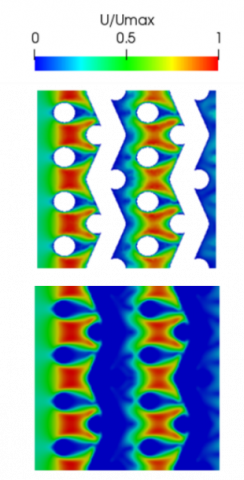Figure 13. Comparison between the classic CFD approach (top) and the porous model (bottom) model, normalized velocity on YZ middle plane, Tin=500 K and Twall=300 K, Re=84

In Figure 14, $\phi$ profile vs Re is shown. A reduction of the thermal efficiency with increasing Re is observed and a maximum difference of 3% between the porous and the CFD model is recovered.

Figure 15 shows the temperature profiles along the centerline for Re=84, characterized by four temperature drops in correspondence of the regenerator wires. Although the temperature drops are placed at the same locations for both approaches, the magnitude of the temperature drops shows some discrepancies. However, the outlet fluid temperature is the same as in the CFD approach.

## 14.pngFigure 14. Comparison between the classic CFD approach and the porous model, $\phi$-Re, Tin=500 K and Twall=300 K

## 15.pngFigure 15. Comparison between the classic CFD approach and the porous model, temperature profiles along z-direction, Tin=500 K and Twall=300 K, Re=84

## 16.pngFigure 16. Comparison between the classic CFD approach (top) and the porous model (bottom), temperature contour plots on YZ middle plane, Tin=500 K and Twall=300 K, Re=84

In Figure 16, the temperature contour plots are compared for both models. An overall good agreement is observed, except for a lower temperature value close to the first row of wires with the porous model. Despite this discrepancy observed in the temperature distributions, the temperature difference between inlet and outlet, as well as the overall thermal performance, is comparable for the two approaches.

The porous model requires a computational time which is about ten times lower than that related to the CFD model. Even though the numerical mesh used with the porous model consists of a higher number of cells than the CFD case (2,025,000 vs 1,523,969 cells), the steady solution is reached in about 800 iterations with the porous model, whereas about 10,000 iterations are needed with the compressible model.

4. Conclusions

In this work, a novel numerical approach is developed to simulate the regenerator of a Stirling engine as porous media under incompressible assumption, in order to compute its performances. Besides, the model is validated by comparing the results with those obtained by using a standard CFD approach.

The first part of the work has been addressed to check the accuracy of the incompressible flow assumption under low Reynolds numbers. Pressure loss and thermal efficiency of the regenerator, as well as flow fields and temperature distributions, are computed to assess the regenerator performance. The results show a good agreement between the compressible and incompressible assumptions, even if some differences in the velocity distribution are observed. The second part of the work describes a new numerical approach that simulates the thermo-fluid dynamic behaviour of the regenerator by considering a porous media approximation of the geometry under investigation. Each cell of the domain is characterized by a given value of porosity: the regions located in the wires are modelled as fully solid, whereas the remaining cells are fully fluid. Moreover, an artificial boundary layer is included in order to correctly reproduce the interaction between walls and fluid, and a heat sink is introduced in the regions where the wires are located to accurately evaluate the heat transfer between wires and fluid. A Darcy term in the momentum equation is included to take into account the pressure drop thru the wires and a linear interpolation of the thermal fluid/solid properties is added to the energy equation. Based on the comparison between compressible vs incompressible solutions, the porous model has been implemented under the incompressible assumption.

The results show that the porous model provides a good agreement with a standard CFD model in terms of both friction coefficient and thermal efficiency of the regenerator. Only slight discrepancies are observed in terms of non-dimensional pressure profiles, and the total pressure drop is comparable. Some differences are observed in terms of temperature profiles, near the first row of wires. However, the heat source term is able to provide an overall good accuracy. Last but not least, the porous model ensures a significant reduction of the total computational cost with respect to the standard CFD approach.

Nomenclature
 AHX Matrix walls area, m2 cf Friction coefficient cp Specific heat at constant pressure, J. kg-1. K-1 dh Hydraulic diameter, m he Local heat transfer, W.m-2-K-1 k Thermal conductivity, W.m-1-K-1 L Matrix axial length, m Nu Nusselt number p Pressure, Pa P/D Pitch-to-diameter ratio Pr Prandtl number q Penalty parameter Re Reynolds number SHX Volumetric heat sink, W m-3 T Temperature, K Twires Wires temperature, K umax Ratio between the frontal maximum velocity and the porosity, m.s-1 U Total local heat transfer, W.m-2-K-1 Vtot Wires total volume, m3 Vm Matrix total volume, m3 VHX Volume of the wire matrix, m3 Greek symbols α Inverse permeability, s-1 ε Fluid volume fraction ν Kinematic viscosity, m2s-1 πv Matrix volumetric porosity ρ Density, kg.m-3 Փ Normalised temperature φ Ratio between the surface area and the volume of the matrix, m-1 Subscripts f Fluid m Mixture s Solid
References

 Andersen, S.K., Carlsen, H., Thomsen, P.G. (2006). Numerical study on optimal Stirling engine regenerator matrix designs taking into account the effects of matrix temperature oscillations. Energy Conversion and Management, 47(5): 894-908. https://doi.org/10.1016/j.enconman.2005.06.006

 Tanaka, M., Yamashita, I., Chisaka, F. (1990). Flow and heat transfer characteristics of the Stirling engine regenerator in an oscillating flow. JSME International Journal. Ser. 2, Fluids Engineering, Heat Transfer, Power, Combustion, Thermophysical Properties, 33: 283-289. https://doi.org/10.1299/jsmeb1988.33.2_283

 Gheith, R., Hachem, H., Aloui, F., Nasrallah, S.B. (2015). Experimental and theoretical investigation of Stirling engine heater: Parametrical optimization. Energy Conversion and Management, 105(11): 285-293. https://doi.org/10.1016/j.enconman.2015.07.063

 Costa, S.C., Tutar, M., Barreno, I., Esnaola, J.A., Barrutia, H., Garcı́a, D., González, M.A., Prieto, J.I. (2014). Experimental and numerical flow investigation of Stirling engine regenerator. Energy, 72(8): 800-812. https://doi.org/10.1016/j.energy.2014.06.002

 Costa, S.C., Barrutia, H., Esnaola, J.A., Tutar, M. (2014). Numerical study of the heat transfer in wound woven wire matrix of a Stirling regenerator. Energy Conversion and Management, 79(3): 255-264. https://doi.org/10.1016/j.enconman.2013.11.055

 Barreno, I., Costa, S.C., Cordon, M., Tutar, M., Urrutibeascoa, I., Gomez, X., Castillo, G. (2015). Numerical correlation for the pressure drop in Stirling engine heat exchangers. International Journal of Thermal Sciences, 97(11): 68-81. https://doi.org/10.1016/j.ijthermalsci.2015.06.014

 Faruoli, M., Viggiano, A., Magi, V. (2018). An investigation of thermo-fluid dynamic performance of a Stirling engine regenerator by means of OpenFOAM. Modelling, Measurement and Control B, 87(9): 151-158. https://doi.org/10.18280/mmc_b.870306

 Bello, F., Viggiano, A., Fanelli, E., Magi, V. (2014). A CFD analysis of the air flow through the matrix regenerator of Stirling engines. ISEC Conference Proceedings, pp. 58-71.

 Buonomo, B., Pasqua, A.D.I., Ercole, D., Manca, O. (2018). Porosity effect on thermal and fluid dynamic behaviors of a compact heat exchanger in aluminum foam. Revue des Composites et des Matériaux Avancés, 28(9): 305-322. https://doi.org/10.1088/1742-6596/745/3/032141

 Costa, S.C., Barreno, I., Tutar, M., Esnaola, J.A., Barrutia, H. (2015). The thermal non-equilibrium porous media modelling for CFD study of woven wire matrix of a Stirling regenerator. Energy Conversion and Management,  89(1): 473-483. https://doi.org/10.1016/j.enconman.2014.10.019

 Mahkamov, K. (2006). Design improvements to a biomass Stirling engine using mathematical analysis and 3D CFD modeling. Journal of Energy Resources Technology, 128: 203-215. https://doi.org/10.1115/1.2213273

 Faruoli, M., Viggiano, A., Magi, V. (2019). A porous media numerical approach for the simulation of stirling engine regenerators. TECNICA ITALIANA-Italian Journal of Engineering Science, 63(2-4): 291-296. https://doi.org/10.18280/ti-ijes.632-425

 Othmer, C. (2008). A continuous adjoint formulation for the computation of topological and surface sensitivities of ducted flows. International Journal for Numerical Methods in Fluids, 58(11): 861-877. https://doi.org/10.1002/fld.1770

 Borrvall, T., Petersson, J. (2002). Topology optimization of fluids in Stokes flow. International Journal for Numerical Methods in Fluids, 41: 77-107. https://doi.org/10.1002/fld.426

 Nield, D.A., Bejan, A. (2013). Convection in Porous Media. 4th ed., New York: Springer Science+Business Media.

 Ushakov, P.A. (1979). Problems of Heat Transfer in Cores of Fast Breeder Reactor, Heat Transfer and Hydrodynamics of Single-phase Flow in Rod Bundles. Izd. Nauka, Leningrad, Russia.